Plot central (quantile-based) posterior interval estimates from MCMC draws. See the Plot Descriptions section, below, for details.

mcmc_intervals(
x,
pars = character(),
regex_pars = character(),
transformations = list(),
...,
prob = 0.5,
prob_outer = 0.9,
point_est = c("median", "mean", "none"),
outer_size = 0.5,
inner_size = 2,
point_size = 4,
rhat = numeric()
)

mcmc_areas(
x,
pars = character(),
regex_pars = character(),
transformations = list(),
...,
area_method = c("equal area", "equal height", "scaled height"),
prob = 0.5,
prob_outer = 1,
point_est = c("median", "mean", "none"),
rhat = numeric(),
border_size = NULL,
bw = NULL,
kernel = NULL,
n_dens = NULL
)

mcmc_areas_ridges(
x,
pars = character(),
regex_pars = character(),
transformations = list(),
...,
prob_outer = 1,
prob = 1,
border_size = NULL,
bw = NULL,
kernel = NULL,
n_dens = NULL
)

mcmc_intervals_data(
x,
pars = character(),
regex_pars = character(),
transformations = list(),
...,
prob = 0.5,
prob_outer = 0.9,
point_est = c("median", "mean", "none"),
rhat = numeric()
)

mcmc_areas_data(
x,
pars = character(),
regex_pars = character(),
transformations = list(),
...,
prob = 0.5,
prob_outer = 1,
point_est = c("median", "mean", "none"),
rhat = numeric(),
bw = NULL,
kernel = NULL,
n_dens = NULL
)

mcmc_areas_ridges_data(
x,
pars = character(),
regex_pars = character(),
transformations = list(),
...,
prob_outer = 1,
prob = 1,
bw = NULL,
kernel = NULL,
n_dens = NULL
)

## Arguments

x

An object containing MCMC draws:

• A 3-D array, matrix, list of matrices, or data frame. The MCMC-overview page provides details on how to specify each these.

• A draws object from the posterior package (e.g., draws_array, draws_rvars, etc.).

• An object with an as.array() method that returns the same kind of 3-D array described on the MCMC-overview page.

pars

An optional character vector of parameter names. If neither pars nor regex_pars is specified then the default is to use all parameters. As of version 1.7.0, bayesplot also supports 'tidy' parameter selection by specifying pars = vars(...), where ... is specified the same way as in dplyr::select(...) and similar functions. Examples of using pars in this way can be found on the Tidy parameter selection page.

regex_pars

An optional regular expression to use for parameter selection. Can be specified instead of pars or in addition to pars. When using pars for tidy parameter selection, the regex_pars argument is ignored since select helpers perform a similar function.

transformations

Optionally, transformations to apply to parameters before plotting. If transformations is a function or a single string naming a function then that function will be used to transform all parameters. To apply transformations to particular parameters, the transformations argument can be a named list with length equal to the number of parameters to be transformed. Currently only univariate transformations of scalar parameters can be specified (multivariate transformations will be implemented in a future release). If transformations is a list, the name of each list element should be a parameter name and the content of each list element should be a function (or any item to match as a function via match.fun(), e.g. a string naming a function). If a function is specified by its name as a string (e.g. "log"), then it can be used to construct a new parameter label for the appropriate parameter (e.g. "log(sigma)"). If a function itself is specified (e.g. log or function(x) log(x)) then "t" is used in the new parameter label to indicate that the parameter is transformed (e.g. "t(sigma)").

Note: due to partial argument matching transformations can be abbreviated for convenience in interactive use (e.g., transform).

...

Currently unused.

prob

The probability mass to include in the inner interval (for mcmc_intervals()) or in the shaded region (for mcmc_areas()). The default is 0.5 (50% interval) and 1 for mcmc_areas_ridges().

prob_outer

The probability mass to include in the outer interval. The default is 0.9 for mcmc_intervals() (90% interval) and 1 for mcmc_areas() and for mcmc_areas_ridges().

point_est

The point estimate to show. Either "median" (the default), "mean", or "none".

inner_size, outer_size

For mcmc_intervals(), the size of the inner and interval segments, respectively.

point_size

For mcmc_intervals(), the size of point estimate.

rhat

An optional numeric vector of R-hat estimates, with one element per parameter included in x. If rhat is provided, the intervals/areas and point estimates in the resulting plot are colored based on R-hat value. See rhat() for methods for extracting R-hat estimates.

area_method

How to constrain the areas in mcmc_areas(). The default is "equal area", setting the density curves to have the same area. With "equal height", the curves are scaled so that the highest points across the curves are the same height. The method "scaled height" tries a compromise between to the two: the heights from "equal height" are scaled using height*sqrt(height)

border_size

For mcmc_areas() and mcmc_areas_ridges(), the size of the ridgelines.

Optional arguments passed to stats::density() to override default kernel density estimation parameters. n_dens defaults to 1024.

## Value

The plotting functions return a ggplot object that can be further customized using the ggplot2 package. The functions with suffix _data() return the data that would have been drawn by the plotting function.

## Plot Descriptions

mcmc_intervals()

Plots of uncertainty intervals computed from posterior draws with all chains merged.

mcmc_areas()

Density plots computed from posterior draws with all chains merged, with uncertainty intervals shown as shaded areas under the curves.

mcmc_areas_ridges()

Density plot, as in mcmc_areas(), but drawn with overlapping ridgelines. This plot provides a compact display of (hierarchically) related distributions.

Other MCMC: MCMC-combos, MCMC-diagnostics, MCMC-distributions, MCMC-nuts, MCMC-overview, MCMC-parcoord, MCMC-recover, MCMC-scatterplots, MCMC-traces

## Examples

set.seed(9262017)

# load ggplot2 to use its functions to modify our plots
library(ggplot2)

# some parameter draws to use for demonstration
x <- example_mcmc_draws(params = 6)
dim(x)
#>  250   4   6
dimnames(x)
#> $Iteration #> NULL #> #>$Chain
#>  "chain:1" "chain:2" "chain:3" "chain:4"
#>
#> \$Parameter
#>  "alpha"   "sigma"   "beta" "beta" "beta" "beta"
#>

color_scheme_set("brightblue")
mcmc_intervals(x)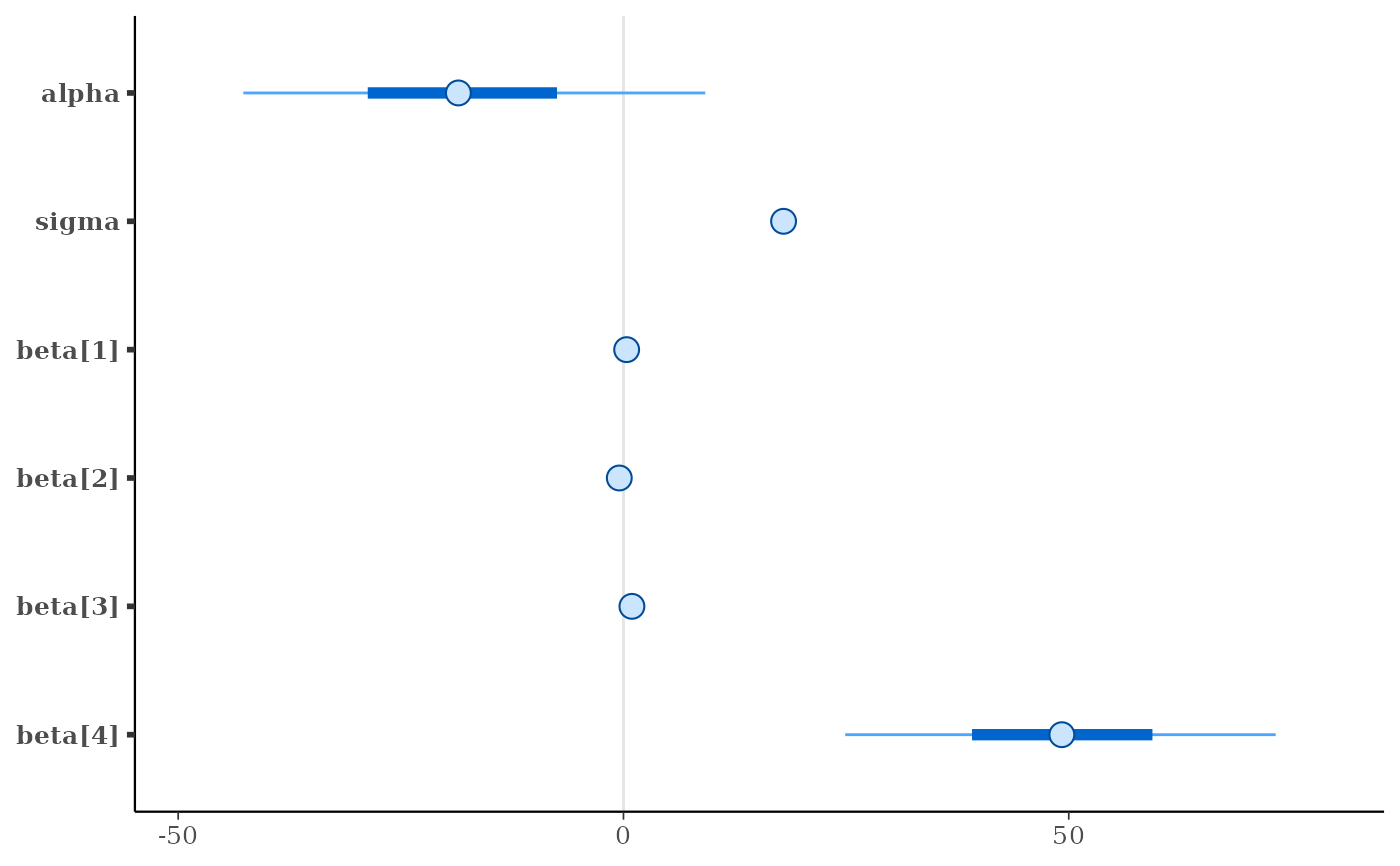mcmc_intervals(x, pars = c("beta", "beta"))mcmc_areas(x, regex_pars = "beta\$[1-3]\$",  prob = 0.8) +
labs(
title = "Posterior distributions",
subtitle = "with medians and 80% intervals"
)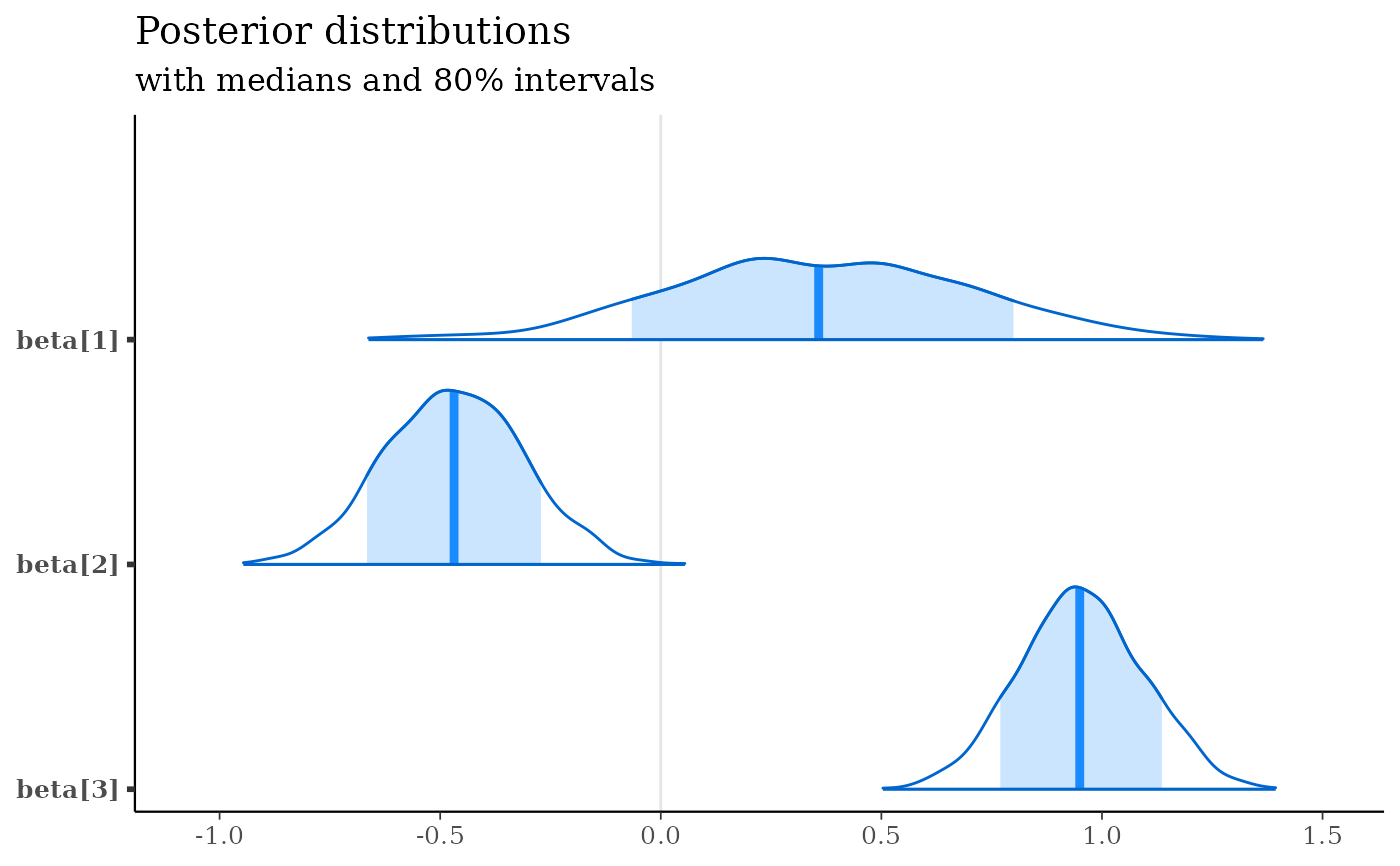color_scheme_set("red")
p <- mcmc_areas(
x,
pars = c("alpha", "beta"),
prob = 2/3,
prob_outer = 0.9,
point_est = "mean",
border_size = 1.5 # make the ridgelines fatter
)
plot(p)# \donttest{
# control spacing at top and bottom of plot
# see ?ggplot2::expansion
p + scale_y_discrete(
limits = c("beta", "alpha"),
expand = expansion(add = c(1, 2))
)
#> Scale for y is already present.
#> Adding another scale for y, which will replace the existing scale.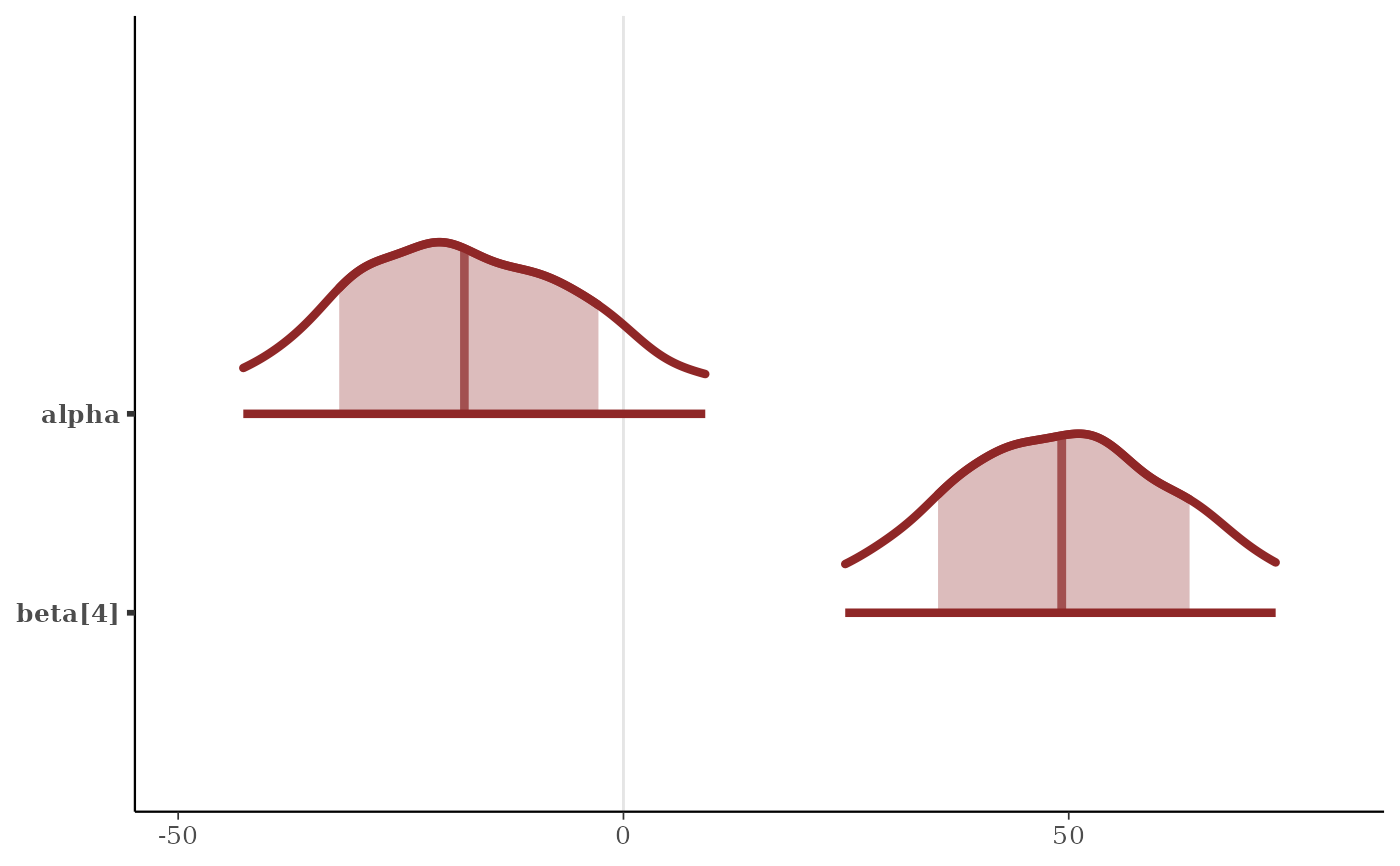p + scale_y_discrete(
limits = c("beta", "alpha"),
expand = expansion(add = c(.1, .3))
)
#> Scale for y is already present.
#> Adding another scale for y, which will replace the existing scale.# relabel parameters
p + scale_y_discrete(
labels = c("alpha" = "param label 1",
"beta" = "param label 2")
)
#> Scale for y is already present.
#> Adding another scale for y, which will replace the existing scale.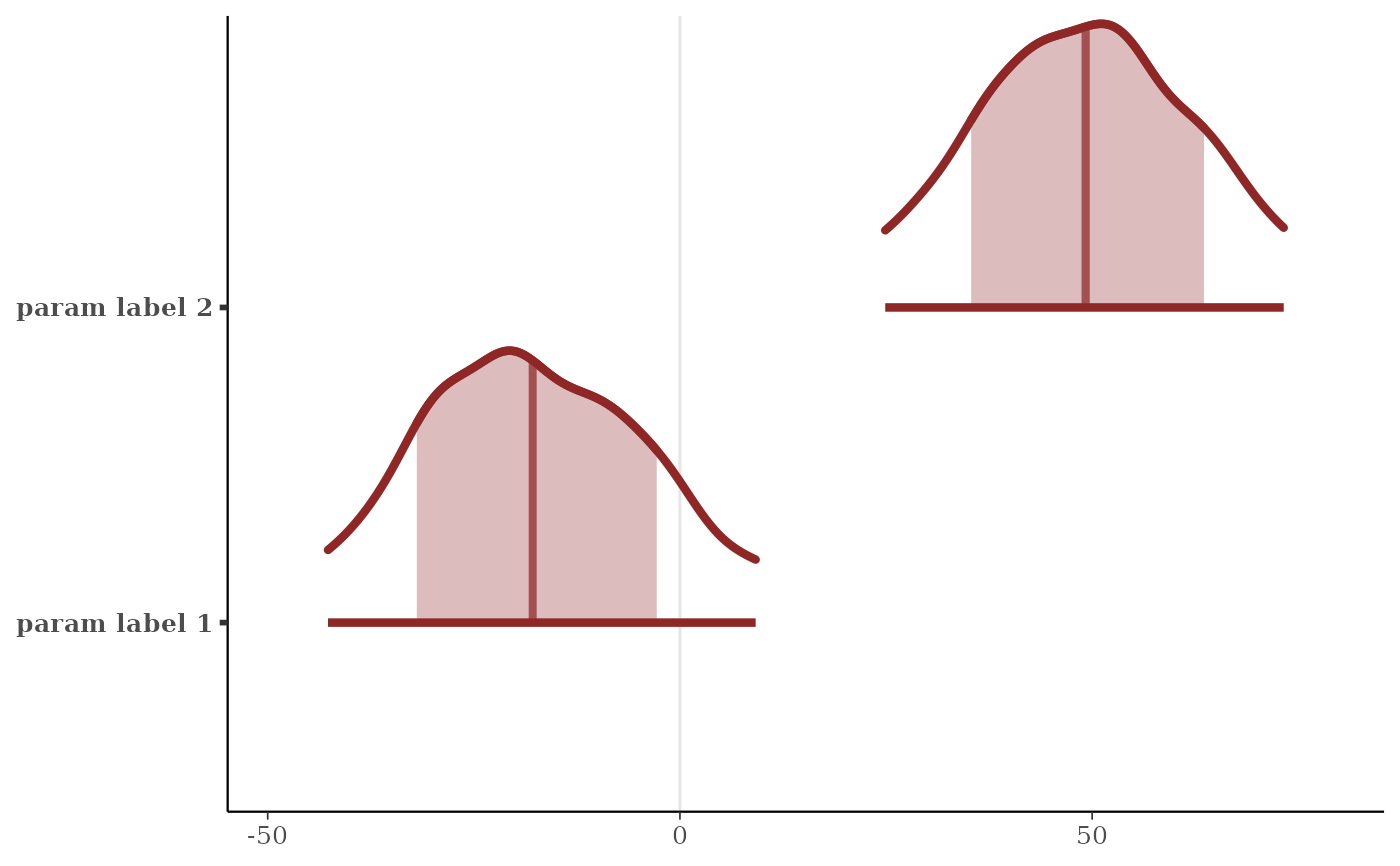# relabel parameters and define the order
p + scale_y_discrete(
labels = c("alpha" = "param label 1",
"beta" = "param label 2"),
limits = c("beta", "alpha")
)
#> Scale for y is already present.
#> Adding another scale for y, which will replace the existing scale.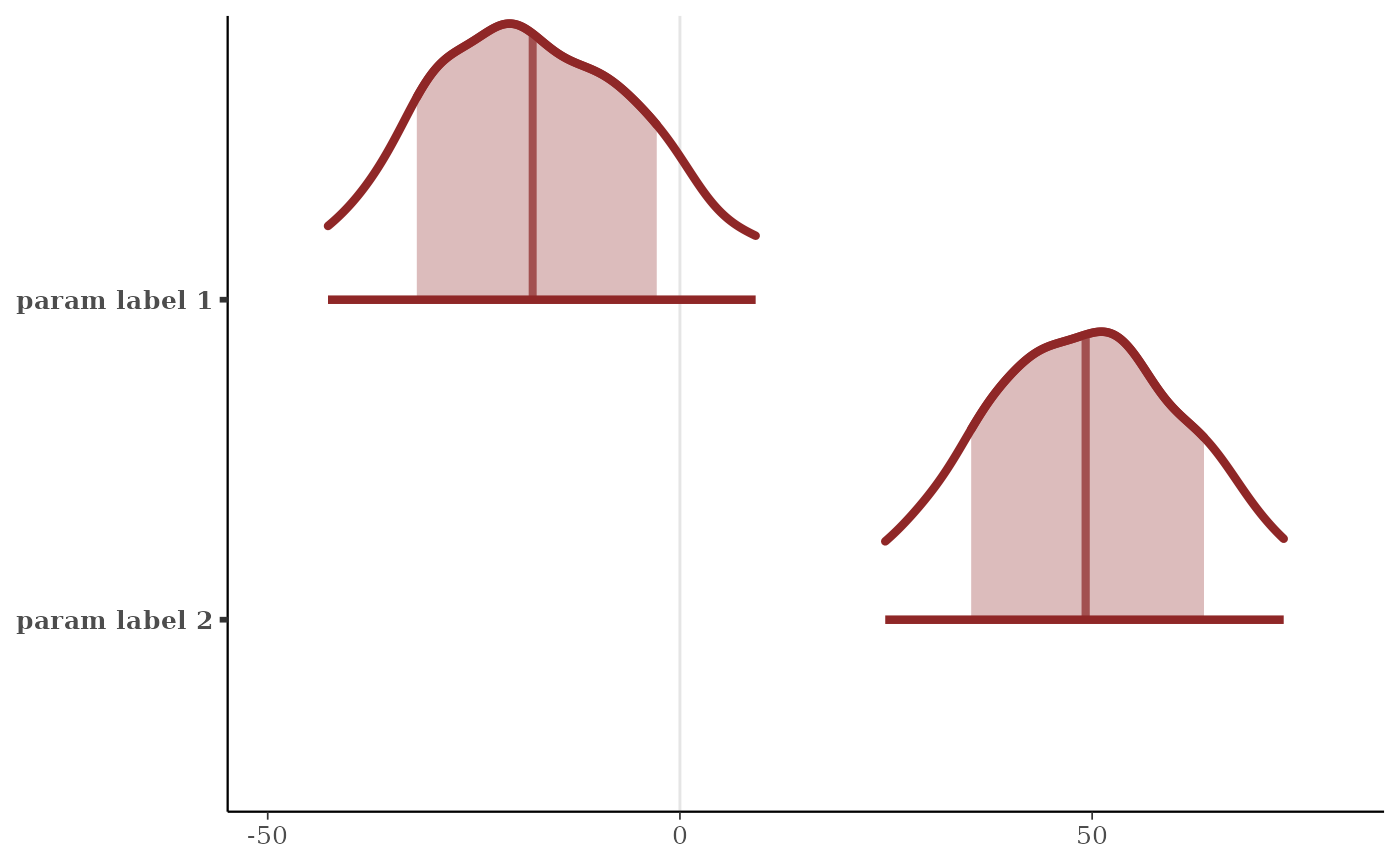# color by rhat value
color_scheme_set("blue")
fake_rhat_values <- c(1, 1.07, 1.3, 1.01, 1.15, 1.005)
mcmc_intervals(x, rhat = fake_rhat_values)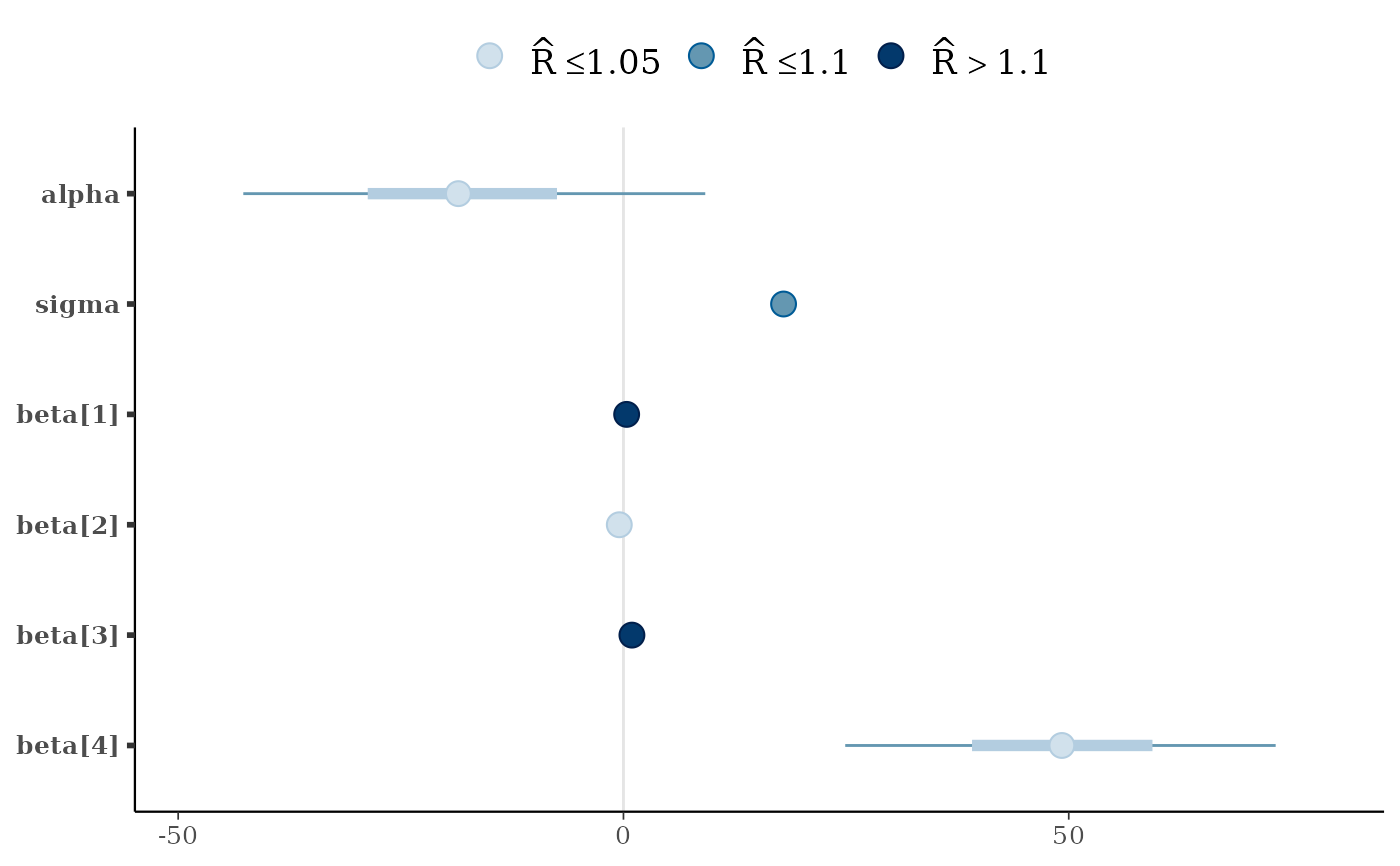# get the dataframe that is used in the plotting functions
mcmc_intervals_data(x)
#> # A tibble: 6 × 9
#>   parameter outer_width inner_wi…¹ point…²      ll       l       m      h     hh
#>   <fct>           <dbl>      <dbl> <chr>     <dbl>   <dbl>   <dbl>  <dbl>  <dbl>
#> 1 alpha             0.9        0.5 median  -42.7   -28.7   -18.5   -7.46   9.18
#> 2 sigma             0.9        0.5 median   17.1    17.6    18.0   18.4   19.0
#> 3 beta           0.9        0.5 median   -0.165   0.136   0.358  0.601  0.916
#> 4 beta           0.9        0.5 median   -0.722  -0.578  -0.469 -0.362 -0.209
#> 5 beta           0.9        0.5 median    0.718   0.858   0.950  1.05   1.19
#> 6 beta           0.9        0.5 median   24.9    39.1    49.2   59.4   73.2
#> # … with abbreviated variable names ¹​inner_width, ²​point_est
mcmc_intervals_data(x, rhat = fake_rhat_values)
#> # A tibble: 6 × 12
#>   parame…¹ outer…² inner…³ point…⁴      ll       l       m      h     hh rhat_…⁵
#>   <fct>      <dbl>   <dbl> <chr>     <dbl>   <dbl>   <dbl>  <dbl>  <dbl>   <dbl>
#> 1 alpha        0.9     0.5 median  -42.7   -28.7   -18.5   -7.46   9.18     1
#> 2 sigma        0.9     0.5 median   17.1    17.6    18.0   18.4   19.0      1.07
#> 3 beta      0.9     0.5 median   -0.165   0.136   0.358  0.601  0.916    1.3
#> 4 beta      0.9     0.5 median   -0.722  -0.578  -0.469 -0.362 -0.209    1.01
#> 5 beta      0.9     0.5 median    0.718   0.858   0.950  1.05   1.19     1.15
#> 6 beta      0.9     0.5 median   24.9    39.1    49.2   59.4   73.2      1.00
#> # … with 2 more variables: rhat_rating <fct>, rhat_description <chr>, and
#> #   abbreviated variable names ¹​parameter, ²​outer_width, ³​inner_width,
#> #   ⁴​point_est, ⁵​rhat_value
mcmc_areas_data(x, pars = "alpha")
#> # A tibble: 2,091 × 7
#>    parameter interval interval_width     x density scaled_density plotting_den…¹
#>    <fct>     <chr>             <dbl> <dbl>   <dbl>          <dbl>          <dbl>
#>  1 alpha     inner               0.5 -28.7  0.0221          0.865          0.865
#>  2 alpha     inner               0.5 -28.7  0.0221          0.865          0.865
#>  3 alpha     inner               0.5 -28.7  0.0221          0.866          0.866
#>  4 alpha     inner               0.5 -28.7  0.0221          0.866          0.866
#>  5 alpha     inner               0.5 -28.6  0.0221          0.867          0.867
#>  6 alpha     inner               0.5 -28.6  0.0221          0.867          0.867
#>  7 alpha     inner               0.5 -28.6  0.0221          0.868          0.868
#>  8 alpha     inner               0.5 -28.6  0.0222          0.868          0.868
#>  9 alpha     inner               0.5 -28.5  0.0222          0.869          0.869
#> 10 alpha     inner               0.5 -28.5  0.0222          0.870          0.870
#> # … with 2,081 more rows, and abbreviated variable name ¹​plotting_density

color_scheme_set("gray")
p <- mcmc_areas(x, pars = c("alpha", "beta"), rhat = c(1, 1.1))
p + legend_move("bottom")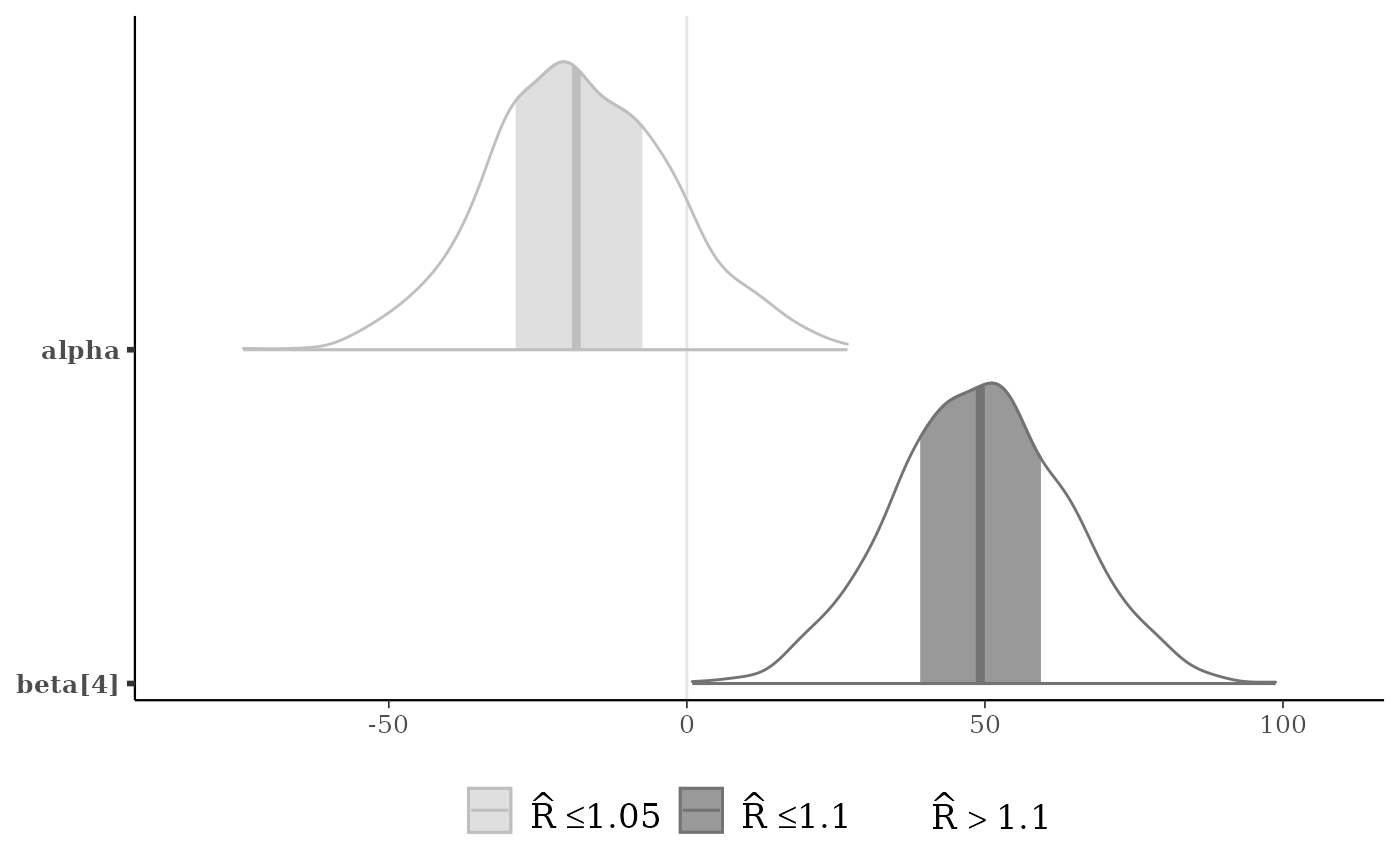p + legend_move("none") # or p + legend_none()# }

# Different area calculations
b3 <- c("beta", "beta", "beta")

mcmc_areas(x, pars = b3, area_method = "equal area") +
labs(
title = "Curves have same area",
subtitle = "A wide, uncertain interval is spread thin when areas are equal"
)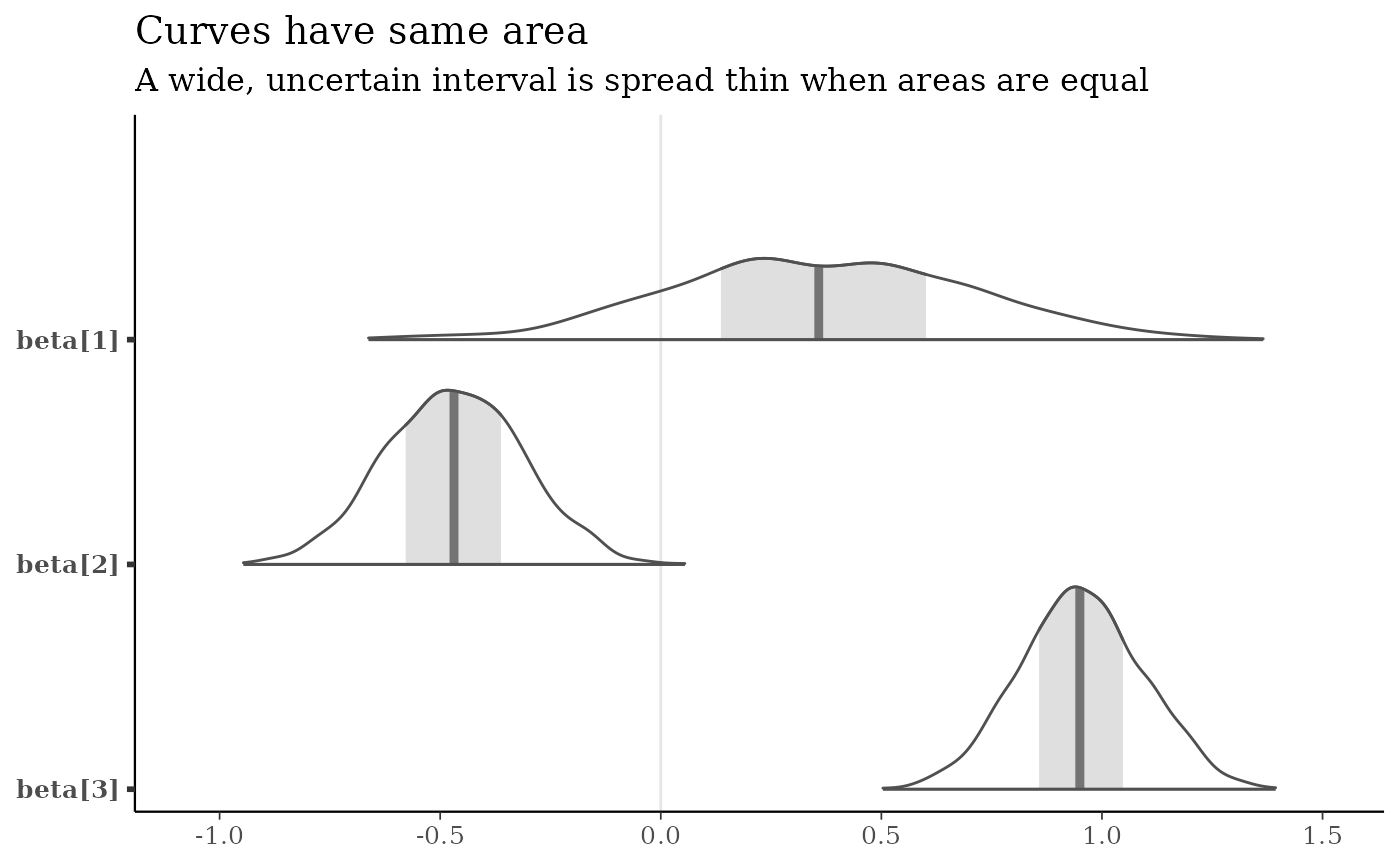mcmc_areas(x, pars = b3, area_method = "equal height") +
labs(
title = "Curves have same maximum height",
subtitle = "Local curvature is clearer but more uncertain curves use more area"
)mcmc_areas(x, pars = b3, area_method = "scaled height") +
labs(
title = "Same maximum heights but heights scaled by square-root",
subtitle = "Compromise: Local curvature is accentuated and less area is used"
)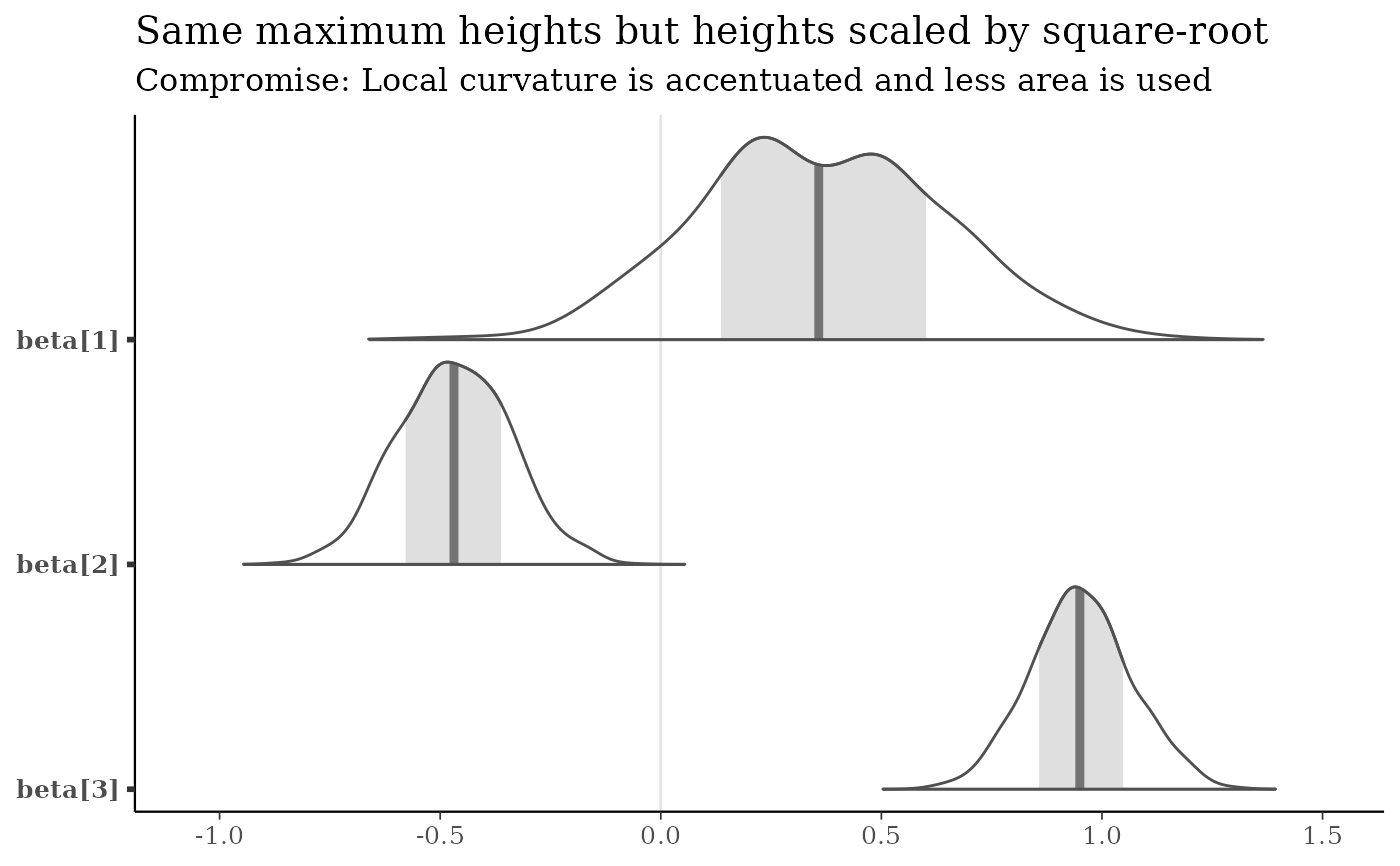# \donttest{
# apply transformations
mcmc_intervals(
x,
pars = c("beta", "sigma"),
transformations = list("sigma" = "log", "beta" = function(x) x + 3)
)# apply same transformation to all selected parameters
mcmc_intervals(x, regex_pars = "beta", transformations = "exp")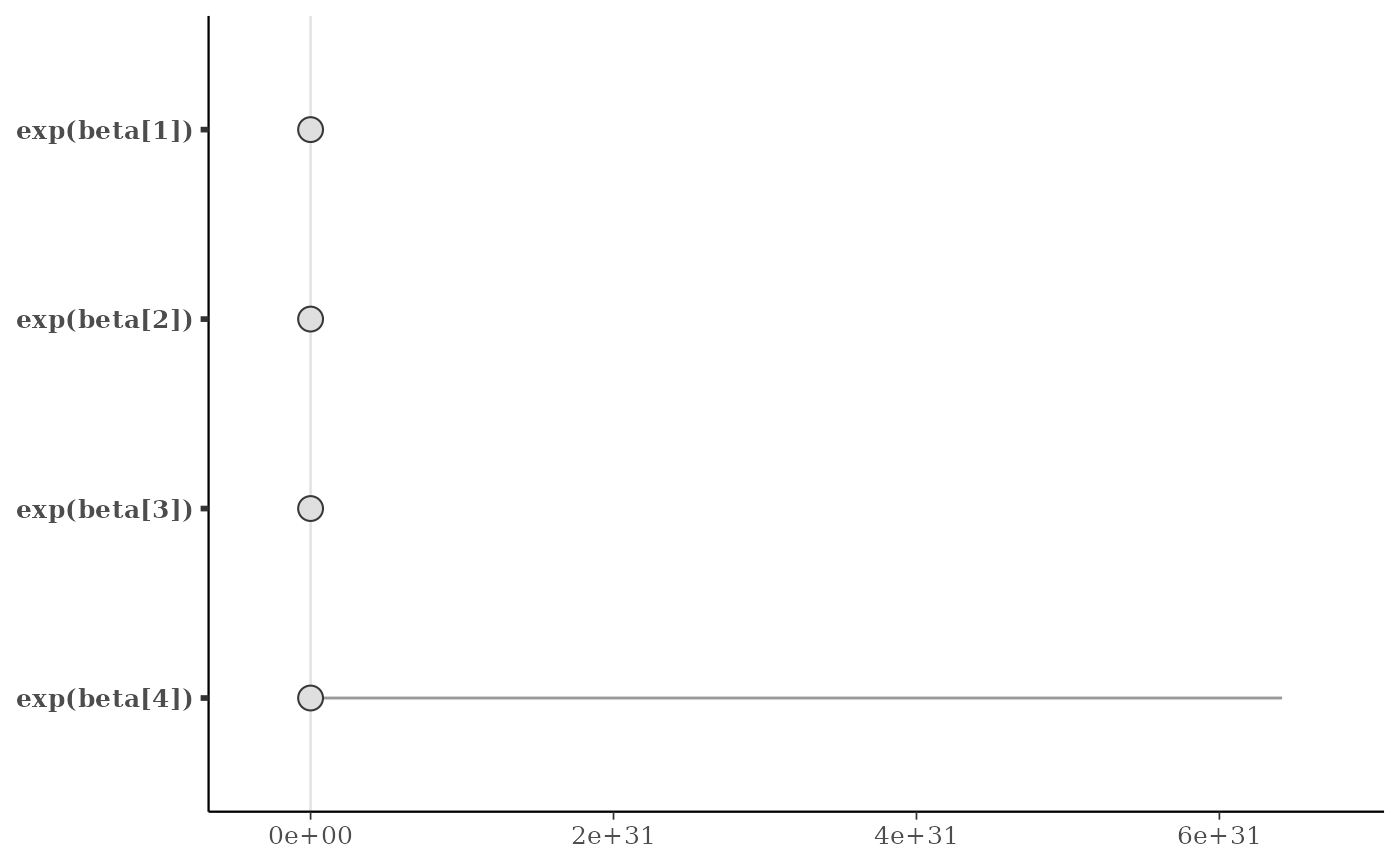# }

# \dontrun{
# example using fitted model from rstanarm package
library(rstanarm)
fit <- stan_glm(
mpg ~ 0 + wt + factor(cyl),
data = mtcars,
iter = 500,
refresh = 0
)
#> Warning: Bulk Effective Samples Size (ESS) is too low, indicating posterior means and medians may be unreliable.
#> Running the chains for more iterations may help. See
#> https://mc-stan.org/misc/warnings.html#bulk-ess
#> Warning: Tail Effective Samples Size (ESS) is too low, indicating posterior variances and tail quantiles may be unreliable.
#> Running the chains for more iterations may help. See
#> https://mc-stan.org/misc/warnings.html#tail-ess
x <- as.matrix(fit)

color_scheme_set("teal")
mcmc_intervals(x, point_est = "mean", prob = 0.8, prob_outer = 0.95)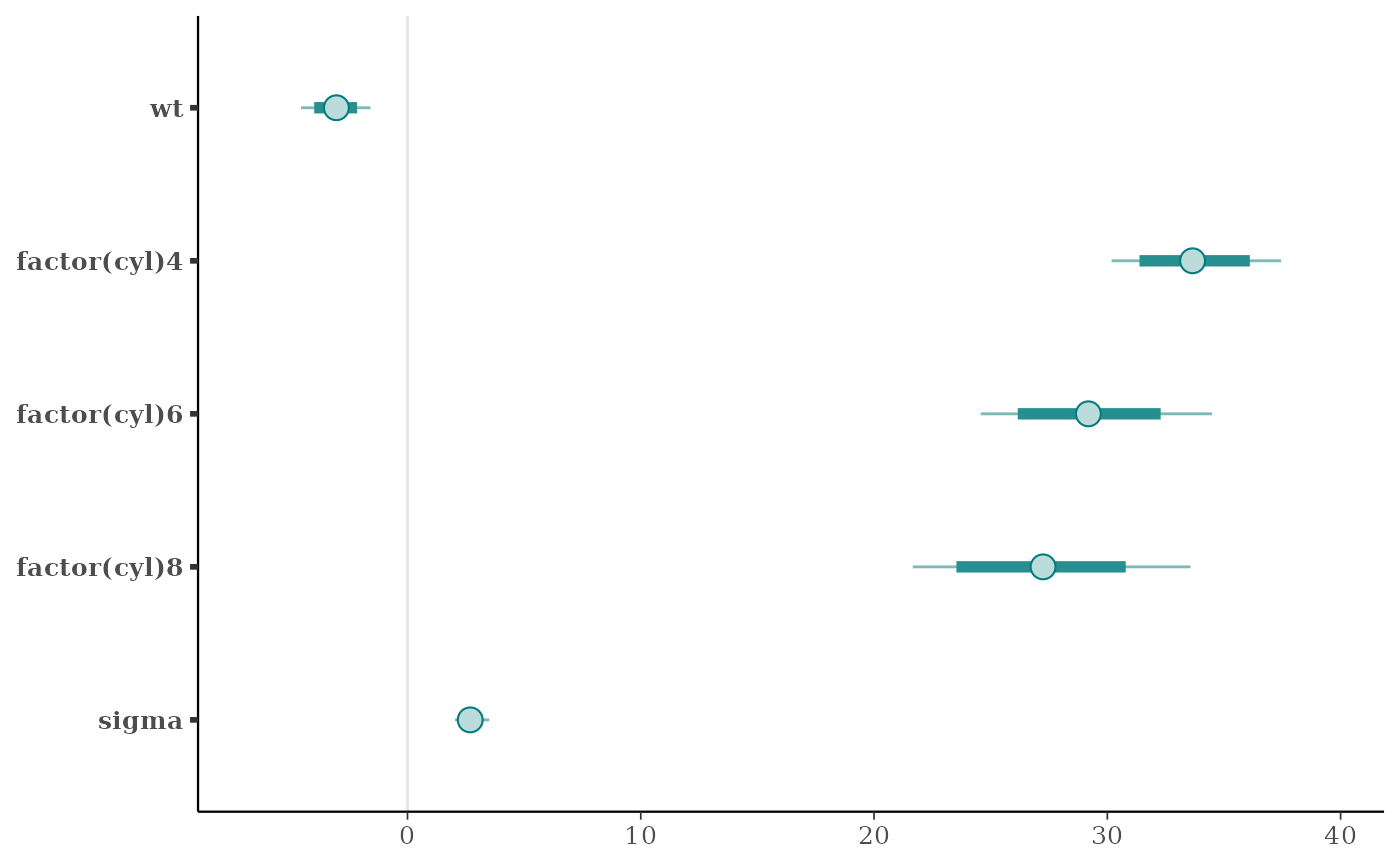mcmc_areas(x, regex_pars = "cyl", bw = "SJ",
rhat = rhat(fit, regex_pars = "cyl"))# }

# \dontrun{
# Example of hierarchically related parameters
# plotted with ridgelines
m <- shinystan::eight_schools@posterior_sample
mcmc_areas_ridges(m, pars = "mu", regex_pars = "theta", border_size = 0.75) +
ggtitle("Treatment effect on eight schools (Rubin, 1981)")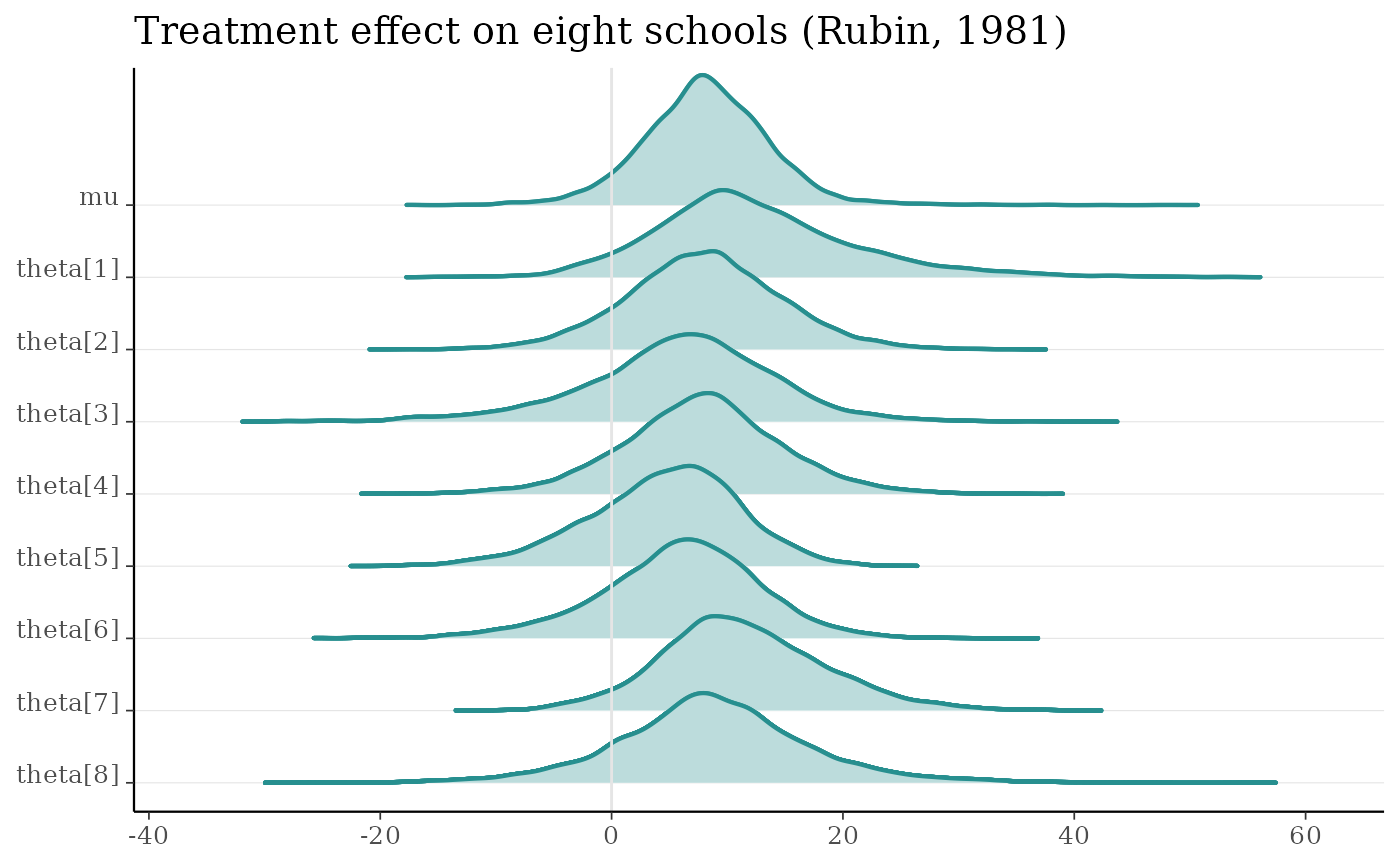# }